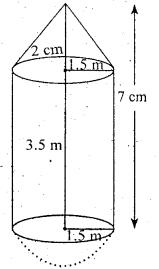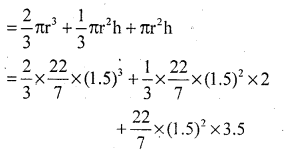# KSEEB Solutions for Class 10 Maths Chapter 15 Surface Areas and Volumes Additional Questions

Students can Download Class 10 Maths Chapter 15 Surface Areas and Volumes Additional Questions, Notes Pdf, KSEEB Solutions for Class 10 Maths helps you to revise the complete Karnataka State Board Syllabus and score more marks in your examinations.

## Karnataka State Syllabus Class 10 Maths Chapter 15 Surface Areas and Volumes Additional Questions

I. Multiple Choice Questions:

Question 1.
A cylindrical pencil sharpened at one edge is the combination of
a. cone and cylinder
b. Frustum of a cone and cylinder
c. A hemisphere and a cylinder
d. two cylinders
a. cone and cylinderQuestion 2.
The curved surface area of a solid hemisphere of radius r is
a. 4πr2
b. 2πr2
c. 4/3πr3
d. 3πr2
b. 2πr2

Question 3.
A shuttlecock used for playing badminton has the shape of a combination of
a. a cone and a sphere
b. frustum of a cone and a hemisphere
c. a cylinder and a hemisphere
d. a cone and a cylinder
b. frustum of a cone and a hemisphere

Question 4.
The ratio of the radii of two spheres is 4 : 5. Find the ratio of their total surface areas is.
a. 5 : 4
b. 4 : 5
c. 16 : 25
d. 25 : 16
c. 16 : 25

Question 5.
If the volume of a cube is 1331 cm3 the length of its edge is equal to
a. 12
b. 11
c. 15
d. 13
b. 11

Question 6.
If the diameter of a sphere is d, then its volume is
a. $$\frac{1}{3}$$πd3
b. $$\frac{1}{24}$$πd3
c. $$\frac{4}{3}$$πd3
d. $$\frac{1}{6}$$πd3
d. $$\frac{1}{6}$$πd3Question 7.
The ratio of the volume of a cube to that of the sphere which will exactly fit inside the cube is
a. 6 : π
b. 4 : π
c. 2 : π
d. 3 : π
a. 6 : π

Question 8.
A cylinder, a cone and hemisphere are of equal base and have the same height the ratio of their volumes is
a. 3 : 2 : 1
b. 2 : 3 : 1
c. 3 : 1 : 2
d. 1 : 2 : 3
c. 3 : 1 : 2

Question 9.
A solid sphere of radius r is melted and cast into the shape of a solid cone of height r. The radius of the base of the cone is.
a. r
b. 3r
c. 4r
d. 2r
d. 2r

Question 10.
If a rectangle revolves about one of its sides and completes a full rotation the solid formed is called
a. sphere
b. cylinder
c. hemisphere
d. cone
b. cylinder

Question 11.
A hollow cylinder has only
a. curved surface area
b. total surface area
c. volume
d. Perimeter
a. curved surface areaQuestion 12.
The formula to find the volume of the cylinder.
a. πr2h
b. 2πr2h
c. 2πr(r + l).
d. πrl
a. πr2h

Question 13.
Is a circular disc is rotated about one of its diameters the solid thus generated is called
a. cylinder
b. sphere
c. cone
d. frustum
b. sphere

Question 14.
The surface area of a sphere is
a. 4πr2
b. 4πr3
c. πrl
d. 2πr (r + 1).
a. 4πr2

Question 15.
The CSA of sphere is
a. 4πr2
b. 3πr2
c. 2πr2
d. πr2
a. 4πr2Question 16.
The surface areas of two sphere are in the ratio 1 : 4 then the ratio of their volumes is
a. 1 : 4
b. 1 : 8
c. 1 : 16
d. 1 : 64
b. 1 : 8

Question 17.
The base radii if two circular cones of the same height are in the ratio 3:5. Find the ratio of their volumes.
a. 3 : 5
b. 5 : 3
c. 9 : 25
d. 27: 125
c. 9 : 25

Question 18.
The volume of a cone of height 4 cm is 168π cm3 the radius of the cone is
a. 6 cm
b. 8 cm
c. 10 cm
d. 12 cm
a. 6 cm

Question 19.
a. 1
b. 2
c. 4
d. 8
d. 8

Question 20.
The volume of a frustum of a cone of height h and ends radii r1 and r2 is.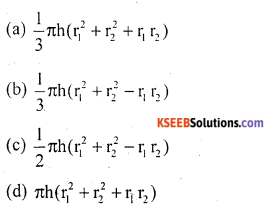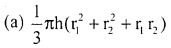Question 21.
If the diameter of a sphere is ‘d’ then its volume is
a. $$\frac{1}{3}$$πd3
b. $$\frac{1}{2}$$πd3
c. $$\frac{4}{3}$$πd3
d. $$\frac{1}{6}$$πd3
d. $$\frac{1}{6}$$πd3Question 22.
The internal and external radii of a hemispherical bowl are r1 and r2 respectively the surface area of the bowl is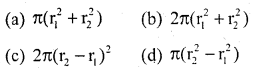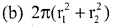Question 23.
The formula to find the slant height of the cone.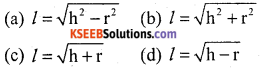b. l = $$\sqrt{h^{2}+r^{2}}$$

Question 1.
Two identical solid hemispheres of equal base radius r cm are struck together along their bases. What will be the total surface area of the combination.
The resultant solid will be a sphere of radius r whose total surface area is 4πr2.

Question 2.
A solid ball is exactly fitted inside the cubical box of side a. What is the volume of the ball?
Diameter of ball = edge of cube = a
∴ r = $$\frac{a}{2}$$
Volume of a ball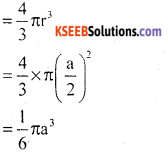Question 3.
The radii of the ends of a frustum of a cone 40 cm high are 20 cm and 11 cm. Find its slant height.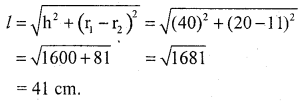Question 4.
A solid piece of iron in the form of a cuboid of dimension 49 cm × 33 cm × 24 cm is melted to form a solid sphere. Find the radius of sphere.
49 × 33 × 24 = 4/3 πr3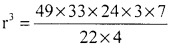r = 21 cmQuestion 5.
Write the formula to find lateral surface area of frustum of cone.
LSA = πl(R + r).

Question 6.
Write the formula to find volume of spherical shell.
Volume of spherical shell = 4/3 π(R3 – r3).

Question 7.
If two cubes of edge 5 cm each are joined end to end, find the surface area of the resulting cuboid.
total length = (l) = 5 + 5 = 10 cm
Breadth = b = 5 cm and
height = h = 5 cm
Surface area = 2 (lb + bh + lh)
= 2 (10 × 5 + 5 × 5 + 5 × 10)
= 2 × 125
= 250 cm2

Question 8.
What is the ratio of the volume of a cube to that of a sphere which will fit inside it.
Let edge of the cube be ‘a’
diameter of the sphere will fit inside the given cube = a
∴ volume of the cube : volume of the sphere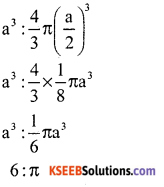Question 9.
Write the formula to find slant height of an frustum cone.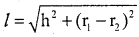Question 1.
A solid hemisphere of wax of radius 12 cm is melted and made into a cone of base radius 6 cm. Calculate the height of the cone.
Radius of the hemisphere, r1 = 12 cm
Radius of the base of the cone, r2 = 6 cm.
Height of the cone = h = ?
volume of wax cone = volume of wax hemisphere.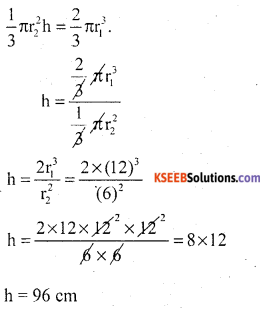Question 2.
21 spheres of equal radii are melted to form a cylinder of radius 14 cm and height 49 cm. Find the radius of sphere.
radius of cylinder = r1 = 14 cm
height h = 49 cm and radius of sphere = r2
Total volume of 21 spheres = volume of cylinder.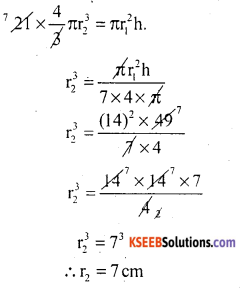∴ radius of the sphere is 7 cm.

Question 3.
A hemispherical bowl of internal radius 9 cm is full of with a liquid, this liquid is to be filled into cylindrical shaped bottles of diameter 3 cm and height 4 cm. How many bottles are necessary to empty the bowl?
hemisphere r1 = 9 cm
Cylinder r2 = $$\frac{d}{2}=\frac{3}{2}$$ cm and h = 4cm
Number of bottles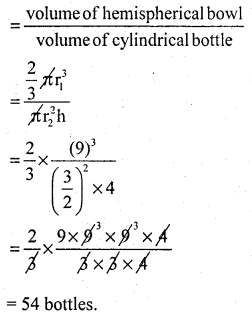Question 4.
A bucket is in the shape of a frustum with the top and bottom circles of radii 15 cm and 10 cm. Its depth is 12 cm. Find its curved surface area and total surface area. (Express the answer in terms of π).
r1 = 15 cm, r2 = 10 cm and h = 12 cm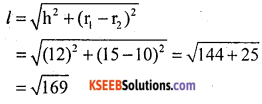l = 13cm
CSA of a bucket = π(r1 + r2)l
= π(15 + 10) 13
= π × 25 × 13
TSA of a bucket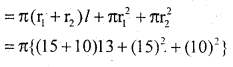= π{25 × 13 + 225 + 100}
= π{325 + 325}
= 650π sq cm

Question 5.
From the top of a cone of base radius 24 cm and height 45 cm, a cone of slant height 45 cm, a cone of slant height 17 cm is cut off. What is the volume of the remaining frustum of cone.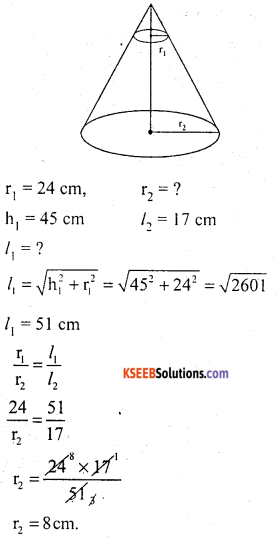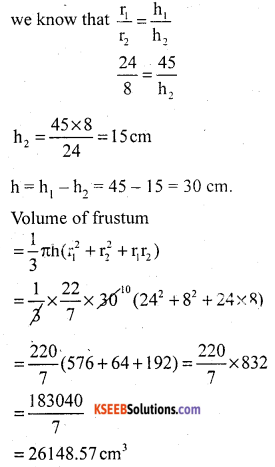Question 6.
A vessel is in the form of a frustum of a cone. Its radius at one end is 8 cm and the height is 14 cm. If its volume is
$$\frac{5676}{3}$$cm3, find the radius of the other end.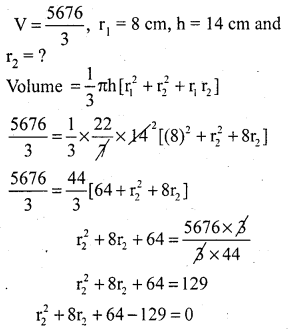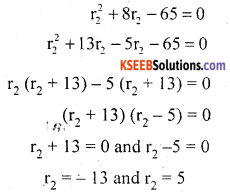∴ Radius of the other end is 5 cm

Question 7.
A solid metallic sphere of diameter 28 cm is melted and recast into a number of smaller cones each of diameter 4$$\frac{2}{3}$$cm and height 3 cm. Find the number of cones so formed.
Diameter of sphere = 28 cm,
radius = r1 = $$\frac{28}{2}$$ = 14cm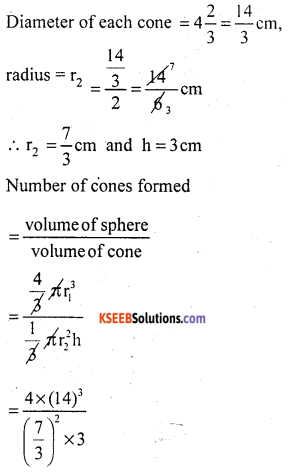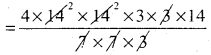= 672 cones.

Question 8.
A petrol tank is in the shape of a cylinder with hemispheres of same radius attached to both ends. If the total length of the tank is 6m and the . radius is 1m, what is the capacity of the tank in litres.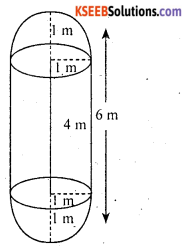Radius of hemisphere = r = 1 m
Radius of cylinder = r = 1 m
height of the cylinder = 4 m
Capacity of the tank = volume of 2 hemispheres + volume of cylinder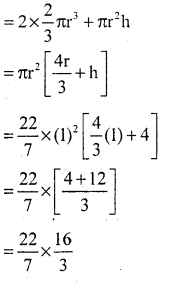= 16.7619 m3. [1 m = 100 cm]
= 16761900 cm3 [1000 cm3 = 1lit]
= $$\frac{16761900}{1000}$$
= 16761.9 litres.

Question 9.
A rocket is in the shape of a cylinder with a cone attached to one end and a hemisphere attached to the other end. All of them are the same radius of 1.5 m. The total length of the rocket is 7m and height of the cone is 2m. Calculate the volume of the rocket.